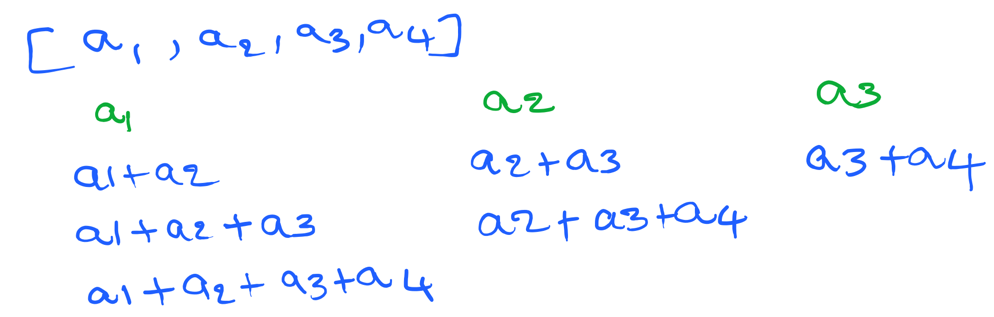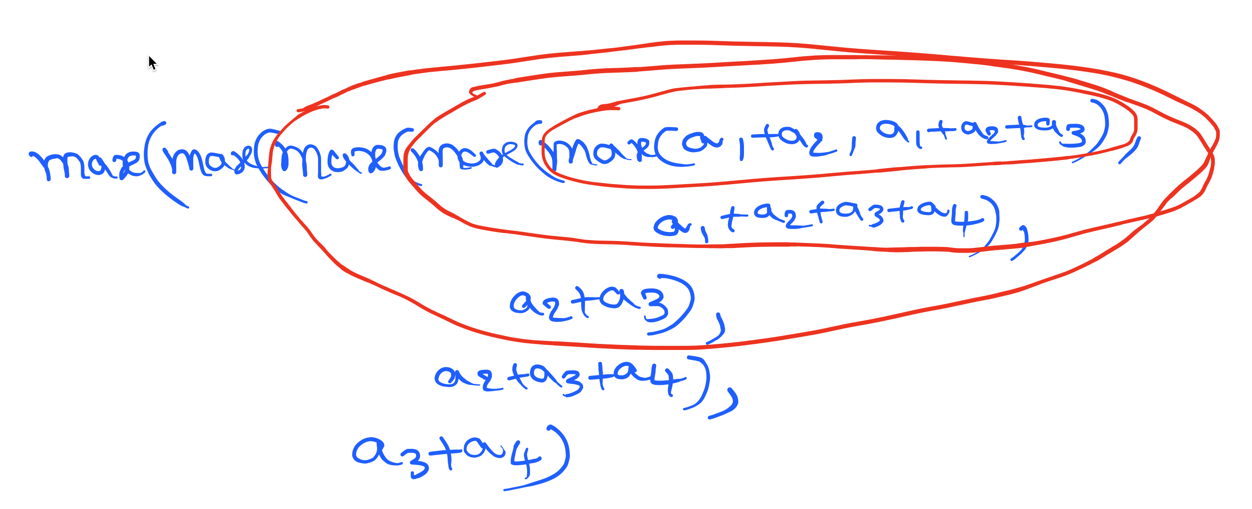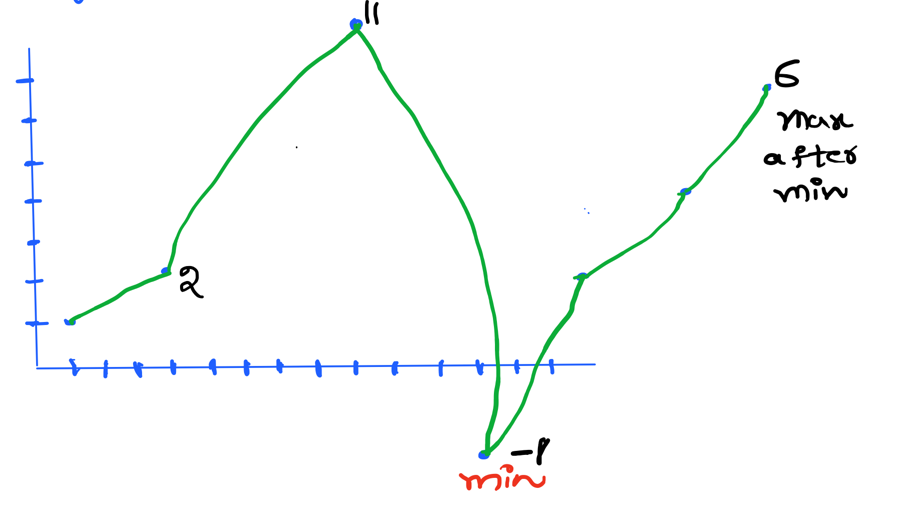Hello everyone! in this post, we’ll discuss about kadane’s algorithm. I’ll try to keep it as simple as possible. The goal of this algorithm is to find a maximum value of sub-array sum. Let’s take an example to understand.

`arr = [7,2,-1,-3,9]`

the maximum sub array sum is = 7+2-1-3+9 = 14

How do we find this ? well, the naive approach is to get all sub-array sum and find the maxmium.

Let’s say we have an array [a1, a2, a3, a4]Now, we get maximum of all i.e. max(a1+a2, a1+a2+a3, a1+a2+a3+a4, a2+a3, a2+a3+a3, a3+a4)

Well, this works but let’s analyze the time complexity. The pseudo code looks like this

``````result = -infinity
for i 0...n
for j in i+1...n
result = max(result, a[i] + a[j])
return result``````

This will give O(n^2) time complexity.

Let’s see how we can optimize thisFrom the above image, we see that there are repeated computations i.e. overlapping sub-problems. If we find the maximum of a1+a2 and a1+a2+a3 gives solution for a sub-problem. We can prove the fact by induction that when combining solutions of all sub-problems will give the overall solution i.e. optimal sub-structure.

From these two facts, we can say that we’re using dynamic programming approach.

The idea is find maximum of all nested sub-problems eventually we’ll reach the solution.As depicted in the above image it is very clearer that `max(a1+a2, a1+a2+a3)` gives local maximum when we do this for all sub-problems we’ll get the global maximum.

at every j, local_max holds maximum sum A[i] + A[i+1] + … A[j-1] for all i ∈ {1…j}. So, `local_max + A[j]` contains next local maximum. By the end we reach n it’ll contain maximum of {1…n-1} elements which gives our result

Let’s write code

``````def find_max_sub_array_sum(arr):
local_max = arr
global_max = float('-inf')
for i in range(1,n):
local_max = max(arr[i], arr[i] + local_max)
global_max = max(local_max, global_max)
return global_max``````

From the problem description, we need to maximize the profit by finding optimal buy and sell price. You cannot sell first and buy later.

Example : [7, 1, 5, 3, 6, 4]

Answer: we’ll buy on day 2 at price 1 and sell on day 5 at price 6 with a total profit of \$5.

When I first saw this problem and above example, I thought of a solution to find the index of minimum element. From that index, traverse the whole array and find the maximum which will give our answer. But, I was wrong. Let’s see howFrom above chart we can see that, the minimum value is -1. After -1, the maximum value is 6. The total profit we get is 7. But, there’s another case priort to minimum which is 2 and 11 which gives profit of 9. This proves that the above approach is wrong.

We need to apply kadane’s algorithm, but with a slight change. Here, we need to find the maximum sub-array sum of stock price differences. Let’s see how this works.

let A be the list of stock prices where A[i] represents stock price on day i.

let’s assume A = [a1, a2, a3, a4]

our aim is to maximize profit which means maximize the difference, let B = [a1, a2-a1, a3-a2, a4-a3]

let’s say a2 and a4 gives the maximum profit, so in B we need to find sum of (a3-a2) + (a4-a3) = a4-a2. Hence, we need to find the largest sub-array sum of price differences

``````def max_profit(prices):
local_max = 0
global_max = 0
for i in range(1, len(prices)):
local_max += (prices[i] - prices[i-1])
local_max = max(0, local_max)
global_max = max(local_max, global_max)
return global_max``````

#### Code Explanation

We can do this in two ways. First, find another array with difference of prices and find maximum sub array. For that we need two iterations and another array. (or) find the difference of prices in the same iteration (refer to line no. 5 in above code). The rest of the code is self-explanatory.

Published Apr 4, 2021

Lokesh Sanapalli is a software engineer who loves to solve real world problems using software engineering principles.